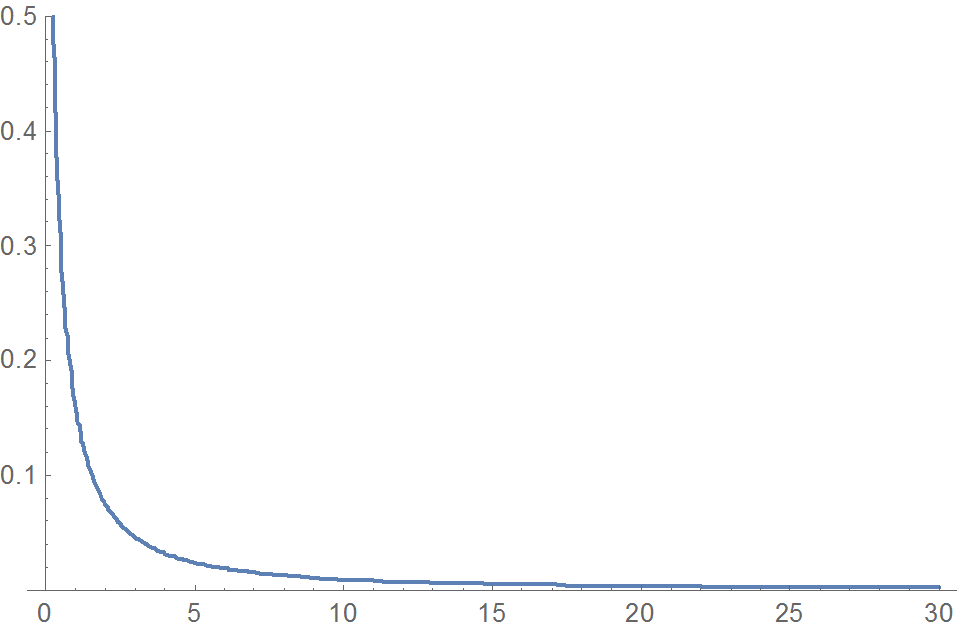29
Sep 16

## Definitions of chi-square, t statistic and F statisticDefinitions of the standard normal distribution and independence can be combined to produce definitions of chi-square, t statistic and F statistic. The similarity of the definitions makes them easier to study.

### Independence of continuous random variables

Definition of independent discrete random variables easily modifies for the continuous case. Let$X,Y$ be two continuous random variables with densities$p_X,\ p_Y$, respectively. We say that these variables are independent if the density$p_{X,Y}$ of the pair$(X,Y)$ is a product of individual densities:

(1)$p_{X,Y}(s,t)=p_X(s)p_Y(t)$ for all$s,t.$

As in this post, equation (1) can be understood in two ways. If (1) is given, then$X,Y$ are independent. Conversely, we if want them to be independent, we can define the density of the pair by equation (1). This definition readily generalizes for the case of many variables. In particular, if we want variables$z_1,...,z_n$ to be standard normal and independent, we say that each of them has density defined here and the joint density$p_{z_1,...,z_n}$ is a product of individual densities.

### Definition of chi-square variableFigure 1. chi-square with 1 degree of freedom

Let$z_1,...,z_n$ be standard normal and independent. Then the variable$\chi^2_n=z_1^2+...+z_n^2$ is called a chi-square variable with$n$ degrees of freedom. Obviously,$\chi^2_n\ge 0$, which means that its density is zero to the left of the origin. For low values of degrees of freedom, the density is not bounded near the origin, see Figure 1.

### Definition of t distributionFigure 2. t distribution and standard normal compared

Let$z_0,z_1,...,z_n$ be standard normal and independent. Then the variable$t_n=\frac{z_0}{\sqrt{(z_1^2+...+z_n^2)/n}}$ is called a t distribution with$n$ degrees of freedom. The density of the t distribution is bell-shaped and for low$n$ has fatter tails than the standard normal. For high$n$, it approaches that of the standard normal, see Figure 2.

### Definition of F distributionFigure 3. F distribution with (1,m) degrees of freedom

Let$u_1,...,u_n,v_1,...,v_m$ be standard normal and independent. Then the variable$F_{n,m}=\frac{(u_1^2+...+u_n^2)/n}{(v_1^2+...+v_m^2)/m}$ is called an F distribution with$(n,m)$ degrees of freedom. It is nonnegative and its density is zero to the left of the origin. When$n$ is low, the density is not bounded in the neighborhood of zero, see Figure 3.

The Mathematica file and video illustrate better the densities of these three variables.

### Consequences

1. If$\chi^2_n$ and$\chi^2_m$ are independent, then$\chi^2_n+\chi^2_m$ is$\chi^2_{n+m}$ (addition rule). This rule is applied in the theory of ANOVA models.
2.$t_n^2=F_{1,n}$. This is an easy proof of equation (2.71) from Introduction to Econometrics, by Christopher Dougherty, published by Oxford University Press, UK, in 2016.